﻿ Empirical Test of the Rational Expectations-permanent Income Hypothesis: Abel and Mishkin’s General Approach: Evidence from AlgeriaPublications are Open
Access in this journal
Article Versions
Export Article
Cite this article
• Normal Style
• MLA Style
• APA Style
• Chicago Style
Research Article
Open Access Peer-reviewed

### Empirical Test of the Rational Expectations-permanent Income Hypothesis: Abel and Mishkin’s General Approach: Evidence from Algeria

Tarek DJEDDI , Tayeb LOUAFI, Said BRIKA
International Journal of Econometrics and Financial Management. 2017, 5(2), 33-36. DOI: 10.12691/ijefm-5-2-2
Published online: July 05, 2017

### Abstract

The purpose of this paper is to test through an empirical analysis the Rational Expectations-Permanent Income Hypothesis. To do this, we referred to the General approach of Abel and Mishkin (1983). Our econometric tests are based on annual data over the period [1985-2015] related to households in Algeria. Our results show the rejection of the general approach’s model which leads to the rejection of the Rational Expectations-Permanent Income Hypothesis. As finding, Algerian households do not form rational expectations about their future income.

### 1. Introduction

Since people care about future, macroeconomic theory cannot be discussed without reference to expectations formation. Expectations formation is a central issue in macroeconomics. In the past, the adoption of various ad hoc assumptions about the process of expectation formation has allowed the development of simple macroeconomic model whose dynamic properties might be analyzed 1.

In the last 50 years, much of the research and debate in macroeconomics has been dominated by one particular hypothesis. It is a hypothesis about the way in which people form their expectations of economic variables. The theory is called the Rational Expectations Hypothesis. It has made a major impact on macroeconometrics and changed the way in which expectations are dealt with and has stressed that a requirement for a successful macroeconomic policy is that it does not rely in consistently being unpredicted.

The economic analysis of the determinants of consumption is among the most fundamental relationships in economics. Under this framework, several hypotheses have appeared. The most important of these hypotheses are: the Absolute Income Hypothesis introduced by the pioneer in this field: J, M. Keynes (1936), the Relative Income Hypothesis by: Duesenberry (1949), the Life Cycle Hypothesis by: Ando and Modigliani (1963), and the Permanent Income Hypothesis by Milton Friedman (1957) 2.

After the appearance of Rational Expectations Hypothesis, some theories examining consumption and its determinants knew several important developments, resulted after the inclusion of unexpected variable (rational) in models of those theories. Hall, R.E.(1978) was the leader in this field, by confirming that the process which determine consumption is linked intrinsically with the process which determines the income through expectations about future income, and as results the appearance of the Rational Expectations-Life Cycle hypothesis, and the, Rational Expectations-Permanent Income Hypothesis.

This paper aims to test the Rational Expectations-Permanent Income Hypothesis, under the general approach developed by Abel and Mishkin (1983). Before this approach there were two approaches. The first, developed by Hall (1978) 3 and Flavin (1981), called ‘the forward looking approach’. The second set forth by Davidson and Hendry (1982) 4, called ‘the backward looking approach’ based on error correction mechanism.

This study use annual data from 1985 to 2015. The crucial question is about the way that Algerian households form their expectations about their future income.

The importance of this study is to enrich the empirical side of the rational expectations hypothesis, by the use of econometric techniques to decipher the encrypted codes that are shown by this hypothesis in the process of forming expectations.

### 2. Literature Review

Abel and Mishkin (1983) 5 has developed a general approach by which to integrate all of the expected variables and random shock, because of their extreme importance on the formation of expectations within the Rational Expectations-Permanent Income H hypothesis, as follows:

Assuming that the dependent variable (households’ consumption) responds to the following model: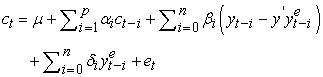(1)

where: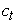: describes the explained endogenous variable (current period’s consumption);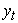: represents the relevant exogenous variable (current period’s disposable income);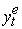: the expectation of.: error term which is normally distributed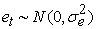.

Thus, the equation (1) represents the reduced form suggests thatis linked to: its previous period’s value, the current period’s disposable income value, and the error of expectations of Income.

But regarding to this equation, we note that it cannot be estimated, because it contains unobservable variables, and to overcome this problem, it is required to determine the mechanism that enables individuals to form their expectations about these unobservable variables.

Abel and Mishkin suggested that the expectations of individuals are rational, and the variableis generated as follow:(2)

where: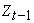: describes the explanatory variables vector, which is supposed to be known by individuals;: parameters vector;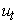: error term (normally distributed and orthogonal on).

The optimal expectation ofwill be given by the mathematical anticipation (by assuming the availability of information at time t-1.) of equation (2) as follows: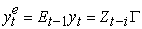(3)

because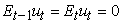.

Thus, and through substitution of (3) in (1) we find the observable reduced form:(4)

The RE-PIH under the general approach’s assumptions, suggests that the vector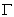that appears in the equation (4) is the same one that came out of the equation (1). However, in practice, it is better to use a generalized formula instead the reduced form which was accessed in the equation (4), as follows:(5)

Where: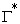is not necessarily equal to.

The joint estimation of the equations (2) and (5), will be the point in which through it we test the rational expectations-permanent income hypothesis in accordance with the general approach proposed by Abel and Mishkin (1983), and that’s through the three following tests (note that the L0 express the logarithmic Maximum Likelihood obtained after this estimation):

• The first test step is interested in evaluating the rational expectations hypothesis, through the estimation of both (2) and (4), with taking into account the assumption that, then compare the ML obtained L1 with L0 through the Likelihood ratio test, where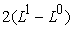is following Chi-squares distribution, with k: the number of restrictions.

• In the second step, we assume that the Rational Expectations Hypothesishas not been rejected, and thus, it will be possible to test the significe effects of the expected variables on the variable(neutrality hypothesis). The test is through comparing the L2 that is obtained from the equation (4) with L1.

• Finally, we investigate the impact of unexpected shocks in a similar method with imposing that:### 3. Methodology

Under the framework of the General approach and our study, equations (2) and (5) had been modified as follows:(6)(7)

where: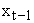: describes explanatory variables matrix, other than disposable income (wealth, for example);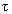: is the coefficients vector associated to the matrix;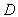: represents the lag operator.

Equation (7) generalizes formulations of both Bilson (1980) 6 and Flavin (1981) 7, The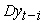has been divided into expected variablesand unexpected variables. In addition, the Equations 6 and 7 gives the possibility to know the impact of the disposable income’s shocks on consumption.

Under this approach, the strong form of the RE-PIH suggests the following restrictions: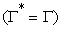; expectations are rational,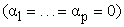; consumption follows a Random walk,; disposable income’s positive chock will affect consumption, because it will incites a revision of permanent income, andbe confined by that revision;; any information contained in previous chocks has been added to the previous period’s disposable income, and should not affect the current consumption;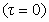; all previous mentioned variables, other than disposable income, should not affect the current consumption.

### 4. Data and Test Procedure

4.1. Data

The data source is from The National Office of Statistics of Algeria, and The National Bank of Algeria, the annual data spans from 1980 to 2015, giving of 36 observations.

The endogenous variable that reflects consumption is represented by the real final consumption of Algerian households instead consumption of impermanent goods, due to the lack of data about it. For the exogenous variable we will express it with the real disposable income. regarding the other explanatory variables, and that contained in the disposable income’s expectational equation, we will use both: the unemployment rate U, the gross domestic product GDPpc, and the urbanization rate URB, because they contribute together in influencing the Algerian household’s consumption (based on a PCA (principal components Analysis) study by the authors).

4.1. Test Procedure

The model subject of estimation and tests take the form of the following equation:(8)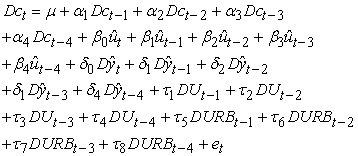(9)

where, equation (8) represents the income expectation model, and equation (9) the consumption model.

For the test of Rational Expectations-Permanent Income Hypothesis it will be as we have mentioned earlier under the framework of the general approach.

### 5. Finding and Discussion

The suggested methodology of the general approach developed by Abel and Mishkin (1983), require the specification of disposable income’s expectation equation, to use it later in the overall consumption’s equation. Theoretically, all variables relevant to this expectation should be included in the matrix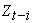.

Based on Dickey-Fuller test and Likelihood Ratio test, only previous period’s disposable income variation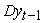, and previous period’s GDP variation, was retained.

After specification of the disposable income’s expectation equation, a Jointestimation process has been conducted using the method of Limited Information Maximum Likelihood LIML, as has been pointed out in Mishkin (1983) 8, and with the assumption that the variation and co-variation matrix of errors is an orthogonal matrix (no serial correlation between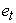and) (This assumption has been criticized by Pudney (1982)).

Table 2 shows the estimation results of the general model, where we note that, for example, the, which represents the effect of the shock of the change in disposable income, show as the most significant parameter in the model, in general the results show a goodness-of-fit of the model.

Table 3 describes results of restriction tests of the RE-PIH according to Abel and Mishkin (1983) approach, which we can extract the following results:

• Like many studies that have used the general approach, the RE-PIH was accepted:• Likelihood Ratio Test of restrictions reject the RE-PIH at α = 0.05 significance level. Also we note that many of explanatory variables, especially the rate of urbanization, appeared as not significant in explaining the household consumption.

• Wald joint test of restrictions shows the rejection of the RE-PIH.

### 6. Conclusion

This study focused on the test of the Rational Expectations-Permanent Income Hypothesis in macroeconometric models, under assumptions of the general approach developed by Abel and Mishkin (1983), case of Algerian households during the period (1980-2015).

The restriction tests chows that the expected and unexpected elements were the main reason for the rejection of RE-PIH.

This study has found that Algerian households do not make rational expectations about their future income.

### References

  David, K. H. BEGG, The rational expectation Revolution in Macroeconomics: theories and evidence, The John Hopkins University Press, Baltimore, Maryland, USA, 1985, 1. In article  Alan, E. H. Speight, Consumption, Rational Expectations and Liquidity: theory and evidence, St. Martin’s Press, New York, USA, 1990. In article PubMed  Hall, R. E, “Stochastic implications of the Life Cycle-Permanent Income Hypothesis: theory and evidence”, Journal of political economy, 86, 971-987, 1978. In article View Article  Davidson. J.E.H. and Hendry, “Interpreting econometric evidence: the behaviour of consumers, expenditure in the UK”, European Economics Review, 16, 177- 192, 1981. In article View Article  Mishkin, F.S, A rational Expectation Approach to Macroeconometrics: Testing policy ineffectiveness and efficient-markets models, The University of Chicago Press, USA, 1983, 17. In article View Article  Bilson, J. F, “The Rational Expectations Approach to the Consumption Function”, European Economics Review, 13, 273-299, 1980. In article View Article  Flavin. M. A, “The adjustment of consumption to changing expectations about future income”, Journal of Political Economy, 98(5), 974-1009, 1981. In article View Article  Abel, A. & Mishkin, F.S, “An integrated view of tests of rationality: market efficiency and short-run neutrality of monetary policy", Journal of monetary economics, 14. 3-24. 1983. In article View ArticleThis work is licensed under a Creative Commons Attribution 4.0 International License. To view a copy of this license, visit http://creativecommons.org/licenses/by/4.0/

### Cite this article:

##### Normal Style
Tarek DJEDDI, Tayeb LOUAFI, Said BRIKA. Empirical Test of the Rational Expectations-permanent Income Hypothesis: Abel and Mishkin’s General Approach: Evidence from Algeria. International Journal of Econometrics and Financial Management. Vol. 5, No. 2, 2017, pp 33-36. http://pubs.sciepub.com/ijefm/5/2/2
##### MLA Style
DJEDDI, Tarek, Tayeb LOUAFI, and Said BRIKA. "Empirical Test of the Rational Expectations-permanent Income Hypothesis: Abel and Mishkin’s General Approach: Evidence from Algeria." International Journal of Econometrics and Financial Management 5.2 (2017): 33-36.
##### APA Style
DJEDDI, T. , LOUAFI, T. , & BRIKA, S. (2017). Empirical Test of the Rational Expectations-permanent Income Hypothesis: Abel and Mishkin’s General Approach: Evidence from Algeria. International Journal of Econometrics and Financial Management, 5(2), 33-36.
##### Chicago Style
DJEDDI, Tarek, Tayeb LOUAFI, and Said BRIKA. "Empirical Test of the Rational Expectations-permanent Income Hypothesis: Abel and Mishkin’s General Approach: Evidence from Algeria." International Journal of Econometrics and Financial Management 5, no. 2 (2017): 33-36.